• 我们提出了一个新的简单的网络框架，即——Transformer，它完全基于注意力机制，丢弃了recurrence和卷convolutions。在两个机器翻译任务上的实验表明，这些模型有着更好的表现，同时具有更高的可并行性，并且所需的...
Attention is all you need
Abstract
主流的序列转换模型基于复杂的递归或卷积神经网络，其中包括一个编码器和一个解码器。表现最好的模型还通过注意力机制连接编码器和解码器。我们提出了一个新的简单的网络框架，即——Transformer，它完全基于注意力机制，丢弃了recurrence和卷convolutions。在两个机器翻译任务上的实验表明，这些模型有着更好的表现，同时具有更高的可并行性，并且所需的训练时间大大减少。我们的模型在WMT 2014 English-to-German翻译任务中达到28.4 BLEU，比包括ensemble在内的现有最佳结果提高了2 BLEU。在WMT 2014 English-to-French翻译任务中，我们的模型在八个GPU上进行了3.5天的训练后，建立了新的单模型最新的BLEU分数41.0，训练成本只占了现有的最好模型中的一小部分。
1 Introduction
尤其是RNN，LSTM，和GRU，已经作为sequence modeling和transduction problems(如语言模型和机器翻译)的最先进的方法而被坚定地建立起来[29,2,5]。此后，人们一直在努力扩大recurrent language models和encoder-decoder体系结构的界限[31、21、13]。

Recurrent models通常沿输入和输出序列的符号位置考虑计算。将位置与计算时间中的步骤对齐，它们会根据先前的隐藏状态$h_{t-1}$和位置$t$的输入生成一系列隐藏状态$h_t$。这种固有的顺序性质阻碍了training examples的并行化，这在较长的序列长度上变得至关重要，因为内存限制限制了示例之间的批处理。最近的工作已经通过factorization tricks和conditional computation在计算效率上取得了显着提高，同时在后者的情况下还提高了模型性能。 但是，顺序计算的基本约束仍然存在。

注意机制已经成为各种任务中compelling sequence modeling和transduction models不可或缺的一部分，允许不考虑其在输入或输出序列中的距离的相关性建模[2,16]。然而，在之外的所有案例中，这种注意机制都与循环网络结合使用。
2 Background
减少顺序计算的目标也构成了Extended Neural GPU 、ByteNet和ConvS2S的基础，它们都使用卷积神经网络作为基本构建块，并行计算所有输入和输出位置的隐藏表示。在这些模型中，从两个任意输入或输出位置关联信号所需的operations随着位置之间的距离增加而增加，ConvS2S为线性，ByteNet为对数。这使得学习远距离位置之间的依赖关系变得更加困难。在Transformer中，这种情况被减少为一个恒定的operations，尽管代价是由于平均注意力加权位置而降低了有效分辨率，正如第3.2节所述，我们用多头注意力抵消了这种影响。

Self-attention（有时称为intra-attention）是一种与单个序列的不同位置相关的注意力机制，目的是计算序列的表示形式。Self-attention已经成功地应用于阅读理解、抽象摘要、文本蕴涵和学习任务无关的句子表征等多种任务中[4,22,23,19]。

端到端的memory networks基于recurrent attention mechanism，而不是sequence-aligned recurrence，并且已被证明在simple-language question answering和language modeling tasks中表现良好。

据我们所知，Transformer是第一个完全依靠self-attention来计算其输入和输出表示的transduction model，而无需使用sequence-aligned RNNs或convolution。在下面的章节中，我们将描述Transformer，motivate self-attention，并讨论它相对于[14,15]和等模型的优势。
3 Model Architecture
大多数具有竞争力的neural sequence transduction models都具有encoder-decoder结构[5,2,29]。编码器将一个符号表示的输入序列$(x_1，\cdots，x_n)$映射到一个连续表示的序列$z = (z_1，\cdots，z_n)$。给定z，则解码器一次生成一个元素的符号的输出序列$(y_1，\cdots，y_m)$。模型的每一步都是auto-regressive，在生成下一个时，会将先前生成的符号作为附加输入。

Transformer遵循此总体架构，对编码器和解码器使用stacked self-attention和point-wise，全连接层，分别如图1的左半部分和右半部分所示。3.1 Encoder and Decoder Stacks
Encoder： 编码器由N = 6个相同的层堆叠组成。 每层有两个子层。第一个子层是multi-head self-attention mechanism，第二个子层是简单的position-wise fully connected feed-forward network。我们在两个子层周围使用一个残差连接，后面进行层归一化，也就是说，每个子层的输出都是LayerNorm(x + Sublayer(x))，其中Sublayer(x)是子层自身实现的函数。为了促进这些残差连接，模型中的所有子层以及嵌入层均产生尺寸为$d_{model}$ = 512的输出。
Decoder： 解码器也由N = 6个相同的层堆叠组成。除了每个编码器层的两个子层之外，解码器插入第三个子层，它对编码器堆栈的输出执行multi-head attention。与编码器类似，我们在每个子层周围使用残差连接，然后进行层归一化。我们还修改了解码器堆栈中的self-attention子层，以防止位置注意到后续位置。这个掩码(masking)，结合输出嵌入偏移一个位置的事实，确保位置i的预测只能依赖于小于i位置的已知输出。

3.2 Attention
An attention function可以描述为将a query和一组key-value对映射到输出，其中query，keys和values都是向量。输出是values的加权和，其中分配给每个value的权重通过query的compatibility function和相应的query计算。
3.2.1 Scaled Dot-Product Attention我们把特别的attention叫作"Scaled Dot-Product Attention" (Figure 2)。输入由$d_k$维的queries和keys，$d_v$维的values组成。我们使用所有keys计算query的点积，除以$\sqrt{d_k}$，然后用softmax函数获得values的权重(如公式一所描述的)。

在实践中，我们同时计算一组queries的attention function，并将其打包成一个矩阵Q。keys和values也被打包成矩阵K和V。输出矩阵由如下的式子计算：
$\operatorname{Attention}(Q, K, V)=\operatorname{softmax}\left(\frac{Q K^{T}}{\sqrt{d_{k}}}\right) V \tag{1}$
两种最常用的attention function是additive attention ，和dot-product (multi-plicative) attention。dot-product attention和我们的算法是类似的，只不过我们的算法多除了一个$\sqrt{d_k}$。Additive attention使用一个带有单一隐含层的feed-forward network来计算compatibility function。虽然这两种方法在理论复杂度上相似，但dot-product attention在实践中速度更快，空间效率更高，因为它可以使用高度优化的矩阵乘法代码实现。当$d_k$值较小时，两种机制的注意表现相似，但当$d_k$值较大时，additive attention优于dot product attention without scaling。我们猜想，当$d_k$的值较大时，点积的大小变大，将softmax函数推入了梯度极小的区域，为了抵消这种影响，我们将点积除以$\sqrt{d_k}$。
3.2.2 Multi-Head Attention
与使用$d_{model}$维的keys、values和queries执行单个attention function相比，我们发现将query、key和value分别用不同的、学到的线性映射h倍到$d_k$、$d_k$和$d_v$维效果更好。基于每个映射版本的query、key和value，我们并行执行attention function，产生$d_v$ 维输出值。将它们连接并再次映射，产生最终值，如图2所示。

Multi-head attention允许模型共同注意来自不同位置的不同表示子空间的信息。用一个单一的attention head，平均抑制这个。
\begin{aligned} \operatorname{MultiHead}(Q, K, V) &=\text { Concat }\left(\text { head }_{1}, \ldots, \text { head }_{\mathrm{h}}\right) W^{O} \\ \text { where head }_{\mathrm{i}} &=\text { Attention }\left(Q W_{i}^{Q}, K W_{i}^{K}, V W_{i}^{V}\right) \end{aligned}
其中的参数矩阵 $W_{i}^{Q} \in \mathbb{R}^{d_{\text {model }} \times d_{k}}, W_{i}^{K} \in \mathbb{R}^{d_{\text {model }} \times d_{k}}, W_{i}^{V} \in \mathbb{R}^{d_{\text {model }} \times d_{v}}$ and $W^{O} \in \mathbb{R}^{h d_{v} \times d_{\text {model }}}$.
在这项工作中，我们采用h = 8 个并行attention层或head。 对每个head，我们使用$d_k$=$d_v$= $d_{model}$/ h = 64。  由于每个head的大小减小，总的计算成本与具有全部维度的单个head attention相似。
3.2.3 Applications of Attention in our Model
Transformer 在三个不同的地方使用了multi-head attention：

在"encoder-decoder attention"层中，query来自前一个解码器层，key和value来自编码器的输出。这允许解码器中的每个位置能关注(attend)到输入序列中的所有位置。这模仿sequence to sequence模型中典型的encoder-decoder的attention机制，例如[38,2,9]。
编码器包含self-attention层。 在self-attention层中，所有的key、value和query来自同一个地方，在这里是编码器中前一层的输出。编码器中的每个位置都可以关注(attend)编码器上一层的所有位置。
类似地，解码器中的self-attention层允许解码器中的每个位置都关注解码器中直到并包括该位置的所有位置。为了保持解码器的自回归属性，我们需要防止解码器中的向左信息流。通过屏蔽softmax的输入中所有不合法连接的值（设置为-∞），我们在scaled dot-product attention中实现。如图2所示。

3.3 Position-wise Feed-Forward Networks
除了attention子层之外，我们的编码器和解码器中的每个层都包含一个fully connected feed-forward network，该前馈网络单独且相同地应用于每个位置。它由两个线性变换组成，之间有一个ReLU激活。
$\operatorname{FFN}(x)=\max \left(0, x W_{1}+b_{1}\right) W_{2}+b_{2}\tag{2}$
尽管线性变换在不同位置上是相同的，但它们每层之间的参数是不同的（不共享参数）。 它的另一种描述方式是两个kernel大小为1的卷积。输入和输出的维度为$d_{model} = 512$，内部层的维度为$d_{ff} = 2048$。
3.4 Embeddings and Softmax
与其他sequence transduction模型类似，我们使用学习到的embedding将输入词符和输出词符转换为维度为$d_{model}$的向量。我们还使用普通的线性变换和softmax函数将解码器输出转换为预测的下一个词符的概率。在我们的模型中，两个embedding层之间和pre-softmax线性变换共享相同的权重矩阵，类似于。在Embedding层中，我们将这些权重乘以$\sqrt{d_{model}}$。
3.5 Positional Encoding
由于我们的模型不包含recurrence和convolution，为了让模型充分利用序列的顺序，我们必须注入序列中关于词符相对或者绝对位置的一些信息。 为此，我们将“位置编码(positional encodings)”添加到编码器和解码器堆栈底部的输入Embedding中。positional encoding和Embedding的维度$d_{model}$相同，所以它们俩可以相加。有多种位置编码可以选择，例如通过学习得到的位置编码和固定的位置编码。
在这项工作中，我们使用不同频率的正弦和余弦函数：
\begin{aligned} P E_{(p o s, 2 i)} &=\sin \left(\operatorname{pos} / 10000^{2 i / d_{\text {model }}}\right) \\ P E_{(\text {pos }, 2 i+1)} &=\cos \left(\text { pos } / 10000^{2 i / d_{\text {model }}}\right) \end{aligned}
其中pos是位置，i是纬度。也就是说，位置编码的每个维度对应于一个正弦曲线。 这些波长形成一个几何级数，从2π 到10000 ⋅ 2π。我们选择这个函数是因为我们假设它允许模型很容易学习对相对位置的关注，因为对任意确定的偏移k, $PE_{pos+k}$可以表示为$PE_{pos}$的线性函数。
我们还使用学习到的位置嵌入进行了试验，发现这两个版本产生几乎相同的结果（参见表3行(E)）。 我们选择了正弦曲线，因为它可以允许模型推断比训练期间遇到的更长的序列。

4 Why Self-Attention
在本节中，我们比较self-attention与recurrent层和convolutional层的各个方面，它们通常用于映射变长的符号序列表示$(x_1,\cdots,x_n)$到另一个等长的序列$(z_1,\cdots,z_n)$，其中$x_i,z_i \in R^d$，例如一个典型的sequence transduction编码器或解码器中的隐藏层。我们使用self-attention是考虑到解决三个问题。

一个是每层计算的总复杂度。 另一个是可以并行的计算量，以所需的最小顺序操作(sequential operation)的数量来衡量。

第三个是网络中长距离依赖之间的路径长度。学习长距离依赖性是许多sequence transduction任务中的关键挑战。 影响学习这种依赖性能力的一个关键因素是前向和后向信号必须在网络中传播的路径长度。输入和输出序列中任意位置组合之间的这些路径越短，学习远距离依赖性就越容易。因此，我们还比较了由不同层类型组成的网络中任意两个输入和输出位置之间的最大路径长度。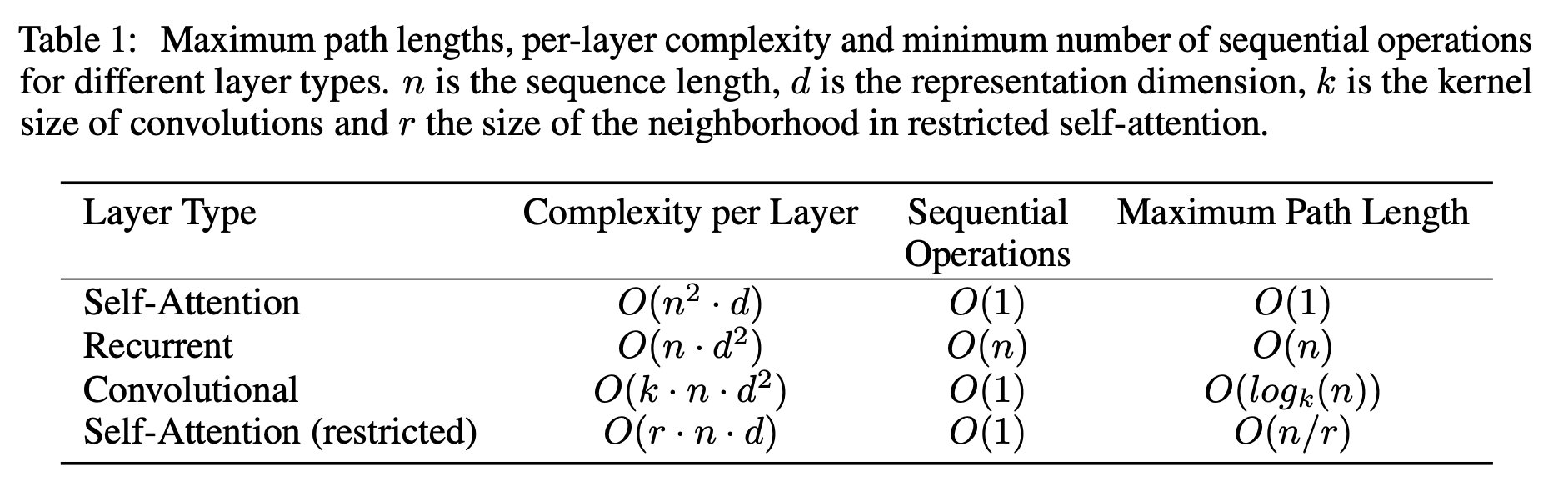如表1所示，self-attention层将所有的位置连接只需要常数数量(O(1))的顺序执行的操作，而recurrent层需要O(n) 顺序操作。在计算复杂性方面，当序列长度n 小于表示维度d 时，self-attention层比recurrent层快，这是机器翻译中最先进的模型中非常常见的情况，例如word-piece，byte-pair表示法。为了提高涉及很长序列的任务的计算性能，可以将self-attention限制在仅考虑大小为r 的邻域内。这会将最大路径长度增加到O(n ∕ r)。 我们计划在未来的工作中进一步调查这种方法。我们计划在未来的工作中进一步调查这种方法。

kernel宽度为k < n的单层卷积不会连接每一对输入和输出的位置。如果要这么做，在邻近核的情况下需要O(n∕k) 个卷积层，在扩展卷积的情况下需要O(logk(n)) 个层，它们增加了网络中任意两个位置之间的最长路径的长度。卷积层通常比循环层花费更大，与因子k有关。然而，可分卷积大幅减少复杂度到O(k ⋅n⋅d + n⋅$d^2$)。 然而，即使k = n，一个可分卷积的复杂度等同于self-attention层和point-wise前向层的组合，即我们的模型采用的方法。

间接的好处是self-attention可以产生更加可解释的模型。我们从我们的模型中研究attention的分布，并在附录中展示和讨论示例。 每个attention head不仅清楚地学习到执行不同的任务，许多似乎展现与句子的句法和语义结构的行为。

自此，本篇论文就翻译到这里了，后面部分不打算翻译了。
论文的下载地址为：https://arxiv.org/pdf/1706.03762.pdf


展开全文nlp attention
• https://www.yiyibooks.cn/yiyibooks/Attention_Is_All_You_Need/index.html
https://www.yiyibooks.cn/yiyibooks/Attention_Is_All_You_Need/index.html


展开全文• ## transformer翻译模型

千次阅读 2019-04-21 23:33:36
transformer google 出品, 基于 encoder-decoder 架构的 seq2seq 自然语言翻译模型. paper 见参考. 创新之处是弃用 RNN,CNN 这样的结构, 采用 self-attention 与 positional-encoding 来简化结构, 加速训练, 并...
google 出品, 基于 encoder-decoder 架构的 seq2seq 自然语言翻译模型. paper 见参考.
创新之处是弃用 RNN,CNN 这样的结构, 采用 self-attention 与 positional-encoding 来简化结构, 加速训练, 并提升效果, 取得了 SOTA 成绩.
BLEU 评测指标
BLEU,  BiLingual Evaluation Understudy , (译作双向语言评估替工) , 一种自动评测机器翻译的方法.
翻译讲究信达雅, 算是主观题. 之所以采用BLEU评估有两点考虑.

人力评估成本大.
便于多个翻译模型之间做对比.

$BLEU\in (0,1)$, 越大表示翻译越精准. 基本思想是 预测结果的 n-gram 与 测试数据的 n-gram 作对比, $n\in \{1,2,3\}$. 看命中效果.
网络结构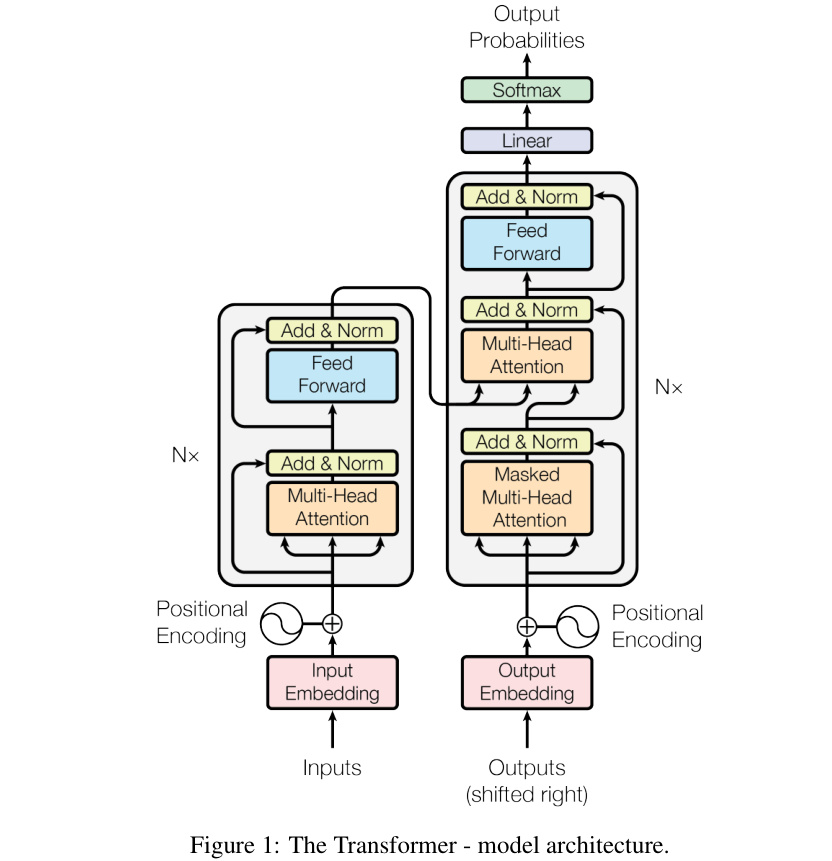self-attention
source 与 target 是两个sequence, self-attention 是指对同一sequence内, 各个token间的关联做计算.
论文中把提出的attention 叫 Scaled Dot-Product Attention.
引入三个共享参数矩阵, Query,Key,Value, 对 embedding 后的token做表达的学习.
所谓attention, 就是这么一个映射关系, $output=f(query, \{key\_value\} )$.
$Attention(Q,K,V)=softmax(\frac{QK^T}{\sqrt{d_k}})V \tag 1$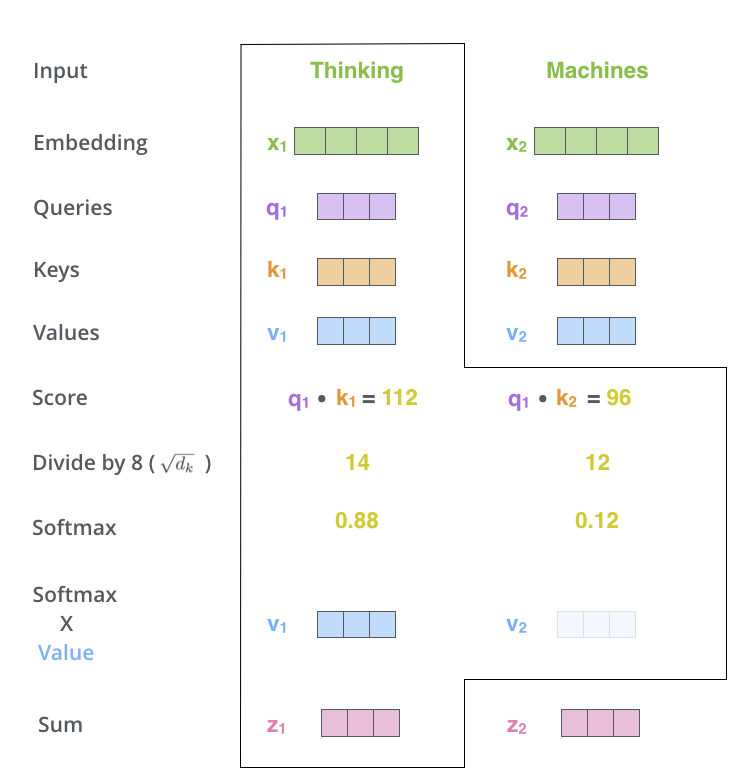图2. $output=f(query, \{key\_value\} )$的直观图示
$MultiHead(Q,K,V)=Concat(head_1,...,head_h)W^O$
where $head_i=Attention(QW_i^Q,KW_i^K,VW_i^V)$, $W^O\in \mathbb R^{hd_v\times d_{model}}$
vanilla attention
除了 source_seq  与 target_seq 各自的 self_attrntion 之外, 还有二者之间的 vanilla_attention. 对应图1 transformer 架构图中, decoder内的第二个 sub-layer. 此时有 vanilla_attention(decoder_Q,encoder_memory_K, encoder_memory_V).
Positional Encoding
将token所在seq的位置信息加以编码表示. 可以是 {sin/cos三角函数, embedding中学习} 两种方案, ablation study 显示差异不大.
mask

future mask
decoder中有个特别点就是masking, masking 的作用就是防止在训练的时候 使用未来时刻才输出的单词。比如训练时，第一个单词是不能参考第二个单词的生成结果的(但可以参考自己)。Masking就会把这个信息变成0，用来保证预测位置 i 的信息只能基于比 i 小的输出。
下面章节的tf实现部分, 会有 下三角矩阵的api说明.

feed-forward
是图片中 feed-forward 的超集, 也包含了 add&norm.
其中 add 残差的操作与时机可以参考
# feed-forward, add & norm
def call(self, inputs, **kwargs):
# Inner layer, ReLU
outputs = Dense(self.num_units, activation=tf.nn.leaky_relu)(inputs)
# Outer layer, linear
outputs = Dense(self.num_units)(outputs)
# Residual connection
outputs += inputs
# Normalize
outputs = utils.modules.layer_norm(outputs)

return outputs

train/predict 不同的 inference
train 阶段, 直接输出整体的 logits #shape=(N, T2, vocab_size) , 与y_ = label_smoothing(tf.one_hot(y, depth=self.hp.vocab_size)) # (N, T2, vocab_size) 计算交叉熵.
在预测阶段, predicts y_hat auto-regressively. 即根据句子Source的中间语义表示C和之前已经生成的历史信息.
$y_i=g(C,y_1,y2_,...,y_{i−1})$
where $C$ 为 source sentence 的语义编码.
tf 实现
官方基于tf, 又搞了 tensor2tensor 库, 内含transformer的实现. github 有人基于tf给出了实现, 见参考 .

数据集
Germany_2_English 翻译任务. 为了单机体验完整流程, 控制数据量. 训练集有 19万 样本, 验证集有 993 条样本. 英德的word加起来构成了 size=8000 的 vocabulary.
超参
$vocab\_size$=32000, $d\_model$=512, $max\_len\_source=100$, $max\_len\_target=100$.
input/output 	xs: tuple of
x: int32 tensor. (N, T1) . T1为当前batch样本的最长原文长度.
x_seqlens: int32 tensor. (N,)
sents1: str tensor. (N,)
ys: tuple of
decoder_input: int32 tensor. (N, T2). T2为当前batch样本的最长译文长度.
y: int32 tensor. (N, T2)
y_seqlen: int32 tensor. (N, )
sents2: str tensor. (N,)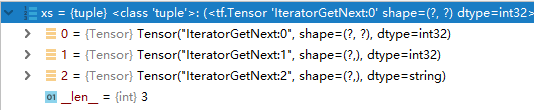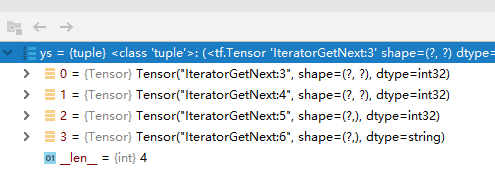主干逻辑

def train(self, xs, ys):
'''
Returns
loss: scalar.
train_op: training operation
global_step: scalar.
summaries: training summary node
'''
# forward
memory, sents1 = self.encode(xs)
logits, preds, y, sents2 = self.decode(ys, memory)

# train scheme
y_ = label_smoothing(tf.one_hot(y, depth=self.hp.vocab_size))
ce = tf.nn.softmax_cross_entropy_with_logits_v2(logits=logits, labels=y_)
nonpadding = tf.to_float(tf.not_equal(y, self.token2idx["<pad>"]))  # 0: <pad>
loss = tf.reduce_sum(ce * nonpadding) / (tf.reduce_sum(nonpadding) + 1e-7)


future mask

diag_vals = tf.ones_like(inputs[0, :, :])  # (T_q, T_k)
# 详见, https://www.tensorflow.org/api_docs/python/tf/linalg/LinearOperatorLowerTriangular?version=stable, 对角线的元素被保留, 不置0.
# 但<=1.4 版本的tf, 是没有这个api的.  可以用 tf.matrix_band_part(input, -1, 0) 替代
tril = tf.linalg.LinearOperatorLowerTriangular(diag_vals).to_dense()  # (T_q, T_k)
masks = tf.tile(tf.expand_dims(tril, 0), [tf.shape(inputs), 1, 1])  # (N, T_q, T_k)

paddings = tf.ones_like(masks) * padding_num
outputs = tf.where(tf.equal(masks, 0), paddings, inputs)

bert
基于双向transformer的预训练语言表达模型.
参考
1.transformer paper, Attention Is All You Need
2. blog, 机器翻译评价指标之BLEU详细计算过程
3. github 非官方实现, transformer
4. blog, 从Encoder-Decoder(Seq2Seq)理解Attention的本质
5. github, bert-use-demo
6. blog, Seq2Seq中的Attention和self-attention
7. my blog, residual connection, 残差链接


展开全文• 通过展示经过机器翻译训练的Levenshtein Transformer可以直接用于自动后期编辑，我们进一步证实了模型的灵活性。 1.介绍 神经序列生成模型已经广泛开发并部署在诸如机器翻译之类的任务中。与会逐步生成字符的最流行...
摘要
现有的神经序列生成模型，要么是从头开始逐步生成字符，要么（迭代地）修改以固定长度为边界的字符序列。在这项工作中，我们提出了Levenshtein Transformer，这是一种新的部分自回归模型，旨在更灵活，更顺畅地生成序列。与以前的方法不同，我们模型的基本操作是insertion和deletion。它们的组合不仅有利于生成，而且有利于优化长度动态变化的序列。我们还提出了一套专门针对它们的新训练技术，由于它们的互补性，可以有效地利用一种作为另一种学习信号。提出的模型在生成（例如机器翻译，文本摘要）和优化任务（例如自动后编辑）方面都达到了相当甚至更好的性能，并且效率大大提高。通过展示经过机器翻译训练的Levenshtein Transformer可以直接用于自动后期编辑，我们进一步证实了模型的灵活性。
1.介绍
神经序列生成模型已经广泛开发并部署在诸如机器翻译之类的任务中。与会逐步生成字符的最流行的自回归模型框架相比，尽管性能略差，但最近的非自回归方法已证明可以在更少的解码迭代次数内执行生成。
在本文中，我们提出了Levenshtein Transformer（LevT），旨在解决当前解码模型缺乏灵活性的问题。值得注意的是，在现有框架中，随着解码的进行，生成的序列的长度是固定的或单调增加的。这仍然与人类的情况不兼容，人类可以修改，替换，撤消或删除其生成的文本的任何部分。因此，LevT被提出通过打破目前的标准化解码机制并将其替换为两个基本操作（insertion和deletion）来弥补这一差距。
我们使用模仿学习训练LevT。结果模型包含两个策略，它们以替代方式执行。从经验上讲，我们显示出LevT在机器翻译和摘要方面达到了比标准Transformer模型可比或更好的结果，同时保持了类似于 (Lee et al., 2018) 的受益于并行解码的效率优势。使用这种模型，我们认为解码变得更加灵活。例如，当给解码器一个空字符时，它会退回到正常的序列生成模型。另一方面，当初始状态是生成的低质量序列时，解码器充当优化模型。实际上，我们证明了通过机器翻译训练的LevT可以直接应用于翻译后编辑，而无需进行任何更改。对于文献中的任何框架，这都是不可能的，因为模型的归纳偏差将生成和完善视为两个不同的任务。
LevT框架中的一个关键组件是学习算法。我们利用insertion and deletion的特征—它们是互补的，但也具有对抗性。我们提出的算法称为“双重策略学习”。这个想法是，当训练一个策略（插入或删除）时，我们将另外一个策略在先前迭代中的输出用作输入。另一方面，绘制了专家策略以提供校正信号。从理论上讲，该学习算法适用于存在双重对抗策略的其他模仿学习场景。在这项工作中，我们主要关注该算法的概念验证，以训练所提出的LevT模型。
为此，我们将贡献总结如下：

我们提出了Levenshtein Transformer（LevT），这是一种由insertion和deletion操作组成的新型序列生成模型。在机器翻译和文本摘要方面，该模型可比强大的Transformer基线获得可比甚至更好的结果，但效率要高得多（就实际机器执行时间而言，最高可提高5倍）；
我们在模仿学习的理论框架下提出了一种相应的学习算法，以解决双重政策的互补性和对抗性；
由于其内置的灵活性，我们认为我们的模型是统一序列生成和优化的先驱尝试。通过这种统一，我们凭经验验证了将机器翻译训练的LevT模型直接应用于翻译后编辑的可行性，而无需进行任何更改。

2.问题定义
2.1 序列生成和优化
通过将它们强制转换为由元组$(\mathcal Y,\mathcal A,\mathcal E,\mathcal R,\textbf y_0)$定义的马尔可夫决策过程（MDP），可以统一序列生成和优化的一般问题。我们考虑由agent与环境$\mathcal E$交互组成的设置，环境$\mathcal E$接收agent的编辑动作并返回修改后的序列。我们将$\mathcal Y=\mathcal V^{N_{max}}$定义为一组长度为$N_{max}$的离散序列，其中$V$是符号词汇。在每次迭代解码中，agent都会收到从头开始或未完成生成的输入$\textbf y$，选择动作$\textbf a$并获得奖赏$r$。我们用$\mathcal A$表示动作集合，用$\mathcal R$表示奖赏函数。通常，奖赏函数$\mathcal R$测量生成序列与真实序列之间的距离$\mathcal R(y)=-\mathcal D(\textbf y,\textbf y^∗)$，该距离可以是任何距离度量，例如Levenshtein距离。将$\textbf y_0∈\mathcal Y$纳入我们的公式至关重要。作为初始序列，当$\textbf y_0$是从另一个系统已经生成的序列时，agent会从本质上学习进行优化，而如果$\textbf y_0$是空序列则会回落到生成。agent通过策略$π$建模，该策略将当前生成映射到$A$上的概率分布。即，$π:\mathcal Y→P(\mathcal A)$。
2.2 Actions: Deletion & Insertion
按照上述MDP公式，根据子序列$\textbf y^k=(y_1,y_2,...,y_n)$，环境调用了两个基本操作（deletion和insertion）来生成$y^{k+1}=E(\textbf y^k,\textbf a^{k+1})$。在这里，我们让$y_1$和$y_n$分别为特殊符号$$和$$。由于我们主要关注单轮生成的策略，因此在本节中为简单起见省略了上标。对于像MT这样的条件生成，我们的策略还包括源信息$\textbf x$的输入，此处也将其省略。
（1）Deletion
deletion策略读取输入序列$\textbf y$，并且对于每个字符$y_i∈\textbf y$，删除策略$π^{del}(d|i,\textbf y)$做出二分类决策，即1（删除字符）或0（保留字符）。我们另外约束$π^{del}(0|1,\textbf y)=π^{del}(0|n,\textbf y)=1$，以避免破坏序列边界。删除分类器也可以看作是GAN中使用的细粒度判别器，在该分类器中，我们为每个预测的字符预测一个“假”或“真实”标签。
（2）Insertion
在这项工作中，构建插入操作要稍微复杂些，因为它涉及两个阶段：占位符预测和字符预测，因此它能够在同一插槽中插入多个字符。首先，在$\textbf y$中所有可能的插槽$(y_i,y_{i+1})$中，$π^{plh}(p|i,\textbf y)$预测添加占位符数目的概率。接下来，对于如上预测的每个占位符，字符预测策略$π^{tok}(t|i,\textbf y)$用词汇表中的实际字符替换占位符。两阶段插入过程也可以看作是Insertation Transformer和屏蔽语言模型的混合体。
（3）Policy combination
回想一下，我们的两个操作是相辅相成的。因此，我们以另一种方式将它们组合在一起。例如，在从空开始的序列生成中，首先调用插入策略，然后将其删除，然后重复执行，直到满足特定的停止条件为止。实际上，可以在这种组合中利用并行性。我们实质上将序列生成器的一个迭代分解为三个阶段：（1）删除字符；（2）插入占位符；（3）用新字符替换占位符。在每个阶段内，所有操作都是并行执行的。更准确地说，给定当前序列$\textbf y=(y_0,...,y_n)$，并假设要进行预测的动作是$\textbf a =\{\underbrace{d_0,...,d_n}_{\textbf d};\underbrace{p_0,...,p_{n-1}}_{\textbf p};\underbrace{t^1_0,...t^{p_0}_0,...,t^{p_{n-1}}_{n-1}}_{\textbf t}\}$，一次迭代的策略是：
$\pi(\textbf a|\textbf y)=\prod_{d_i\in\textbf d}\pi^{del}(d_i|i,\textbf y)\cdot \prod_{p_i\in \textbf p}\pi^{plh}(p_i|i,\textbf y')\cdot \prod_{t_i\in\textbf t}\pi^{tok}(t_i|i,\textbf y''),\tag{1}$
其中$\textbf y'=\mathcal E(\textbf y,\textbf d)$和$\textbf y''=\mathcal E(\textbf y',\textbf p)$。我们在每个子任务中并行化计算。
3.Levenshtein Transformer
在本节中，我们介绍了Levenshtein Transformer的框架和双重策略学习算法。总体而言，我们的模型将字符序列（或空字符）作为输入，然后通过在插入和删除交替进行迭代地修改它，直到两个策略组合在一起为止。我们在附录中描述了详细的学习和推理算法。
3.1 模型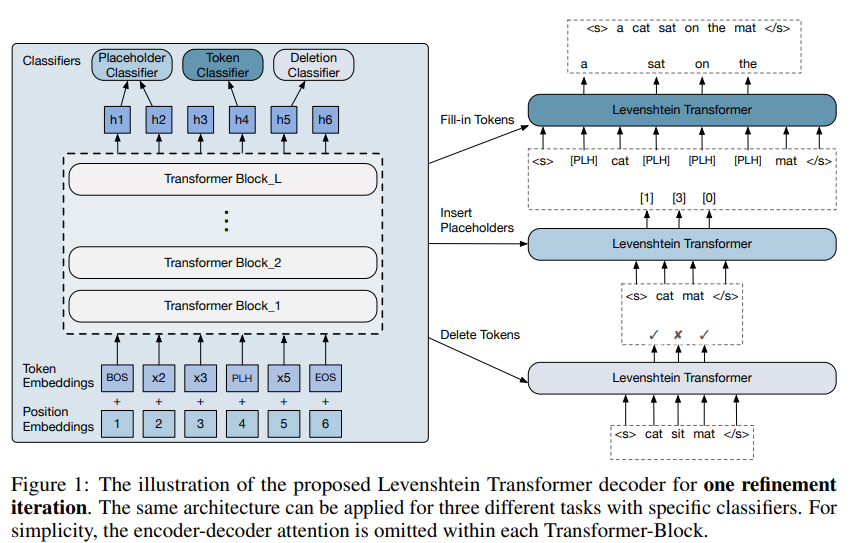我们使用Transformer作为基本构建块。对于条件生成，源$\textbf x$包含在每个TransformerBlock中。第l个块的状态为：
$\textbf h^{(l+1)}_0,\textbf h^{(l+1)}_1,...,\textbf h^{(l+1)}_n=\begin{cases} E_{y_0}+P_0,E_{y_1}+P_1,...,E_{y_n}+P_n, & l=0\\ TransformerBlock_l(\textbf h^{(l)}_0,\textbf h^{(l)}_1,...,\textbf h^{(l)}_n), & l\gt 0 \end{cases}\tag{2}$
其中$E∈\mathbb R^{|V|×d_{model}}$和$P∈\mathbb R^{N_{max}×d_{model}}$分别是字符和位置嵌入。我们展示了提出的用于修改序列（删除，插入）的LevT模型的示意图，如图1所示。
（1）Policy Classifiers
解码器的输出$(\textbf h_0,\textbf h_2,...,\textbf h_n)$传递给三个策略分类器：
Deletion Classifier。LevT扫描输入字符（边界除外），并预测每个字符位置是否删除（0）或保留（1），
$\pi^{del}_{\theta}(d|i,\textbf y)=softmax(\textbf h_i\cdot A^T),i=1,...n-1,\tag{3}$
其中$A∈\mathbb R^{2×d_{model}}$，并且我们总是保留边界字符。
Placeholder Classifier。LevT通过将隐藏表示转换为分类概率分布来预测要在每个连续位置对上插入的字符数量：
$\pi^{plh}_{\theta}(p|i,\textbf y)=softmax(concat(\textbf h_i,\textbf h_{i+1})\cdot B^T),i=0,...n-1,\tag{4}$
其中$B∈\mathbb R^{(K_{max}+1)×(2d_{model})}$。根据它预测的字符数量（0到$K_{max}$），我们在当前位置插入相应的占位符数量。在我们的实现中，占位符由保留在词汇表中的特殊字符$$表示。
Token Classifier。按照占位符预测结果，LevT需要填写字符来替换所有占位符。这是通过训练字符预测器来实现的，如下所示：
$\pi^{tok}_{\theta}(t|i,\textbf y)=softmax(\textbf h_i\cdot C^T),\forall y_i=,\tag{5}$
其中$C∈\mathbb R^{|V|×d_{model}}$是与嵌入矩阵共享的参数。
（2）Weight Sharing
我们的默认实现始终假设这三个操作共享相同的Transformer，以使从其他操作中学到的特征受益。但是，也有可能禁用权重共享并为每个操作训练单独的解码器，这增加了模型的容量，同时不影响整体推理时间。
（3）Early Exit
尽管在上述三个分类器上共享相同的Transformer结构在参数上有效，但是仍有改进的空间，因为一次解码迭代需要网络的三个完整通道。为了在性能和计算成本之间进行权衡，我们提出对$π^{del}$和$π^{plh}$执行early exit（将分类器附加到中间块而不是最后一个块），以减少计算，同时使$π^{tok}$始终基于最后一块，这是因为字符预测通常比其他两个任务更具挑战性。
3.2 3.2 双重策略学习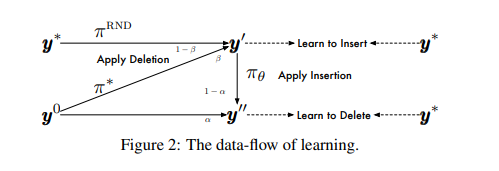（1）Imitation Learning
我们使用模仿学习来训练Levenshtein Transformer。本质上，我们让agent模仿从某些专家策略$π^∗$中得出的动作。专家政策源自直接使用真实目标或通过序列蒸馏过滤后获得的噪声较小的版本。目的是使以下期望最大化：
$\underbrace{\mathbb E_{\textbf y_{del}\sim d_{\tilde \pi_{del}};\textbf d^*\sim \pi^*}\sum_{d^*_i\in\textbf d^*}log~\pi^{del}_{\theta}(d^*_i|i,\textbf y_{del})}_{Deletion~Objective}+\underbrace{\mathbb E_{\textbf y_{ins}\sim d_{\tilde \pi_{ins}};\textbf p^*,\textbf t^*\sim \pi^*}[\sum_{p^*_i\in \textbf p^*}log~\pi^{plh}_{\theta}(p^*_i|i,\textbf y_{ins})+\sum_{t^*_i\in \textbf t^*}log~\pi^{tok}_{\theta}(t^*_i|i,\textbf y'_{ins})]}_{Insertion Objective},$
其中$\textbf y'_{ins}$是在$\textbf y_{ins}$上插入占位符$\textbf p^∗$后的输出。$\tilde π_{del},\tilde π_{ins}$是roll-in策略，我们从它们的诱导状态分布$d_{\tilde π_{del}},d_{\tilde π_{ins}}$反复绘制状态（序列）。这些状态序列首先由专家策略执行，并由专家返回建议的操作，然后将它们的条件对数概率最大化。根据定义，roll-in策略确定训练期间馈入πθ的状态分布。在这项工作中，我们有两种方法来构建roll-in策略—在真实数据中增加噪声或使用对抗政策的输出。图2显示了这种学习范例的示意图。我们正式记录了以下roll-in策略。
Learning to Delete。通过混合因子$α∈[0,1]$，我们将$\tilde π_{del}$设计为初始输入$\textbf y^0$或应用模型的插入操作输出之间的随机混合：
$d_{\tilde \pi_{del}}=\{\textbf y^0~~if~~u\lt \alpha~~else~~\mathcal E(\mathcal E(\textbf y',\textbf p^*),\tilde \textbf t),\textbf p^*\sim\pi^*,\tilde \textbf t\sim \pi_{\theta}\}\tag{6}$
其中$u〜Uniform[0,1]$和$\textbf y'$是准备插入字符的任何序列。通过采样而不是从等式（5）中求出argmax来获得$\tilde \textbf t$。
Learning to Insert。与删除步骤类似，受训练屏蔽语言模型的最新发展启发，我们将删除输出和对真实序列随机丢弃单词所得输出混合使用。我们之所以使用随机丢弃作为加噪的一种形式，以鼓励更多的探索。令$β∈[0,1]$和$u〜Uniform[0,1]$，
KaTeX parse error: Undefined control sequence: \p at position 169: …\textbf d \sim \̲p̲^{RND}\}\tag{7}
（2）Expert Policy
在模仿学习中构建专家策略至关重要，因为学习起来不能太难或太弱。具体来说，我们考虑了两种类型的专家：
Oracle。一种方法是建立一个可访问真实序列的oracle。它通过以下方式返回最佳操作$\textbf a^∗$（插入$p^∗,t^∗$或删除$d^∗$）：
$\textbf a^*=\mathop{argmin}\limits_{\textbf a}~\mathcal D(\textbf y^*,\mathcal E(\textbf y,\textbf a))\tag{8}$
在这里，考虑到可以通过动态规划有效地获得动作建议，我们使用Levenshtein距离作为$\mathcal D$。
Distillation。我们还探索使用另一种teacher模型来提供专家策略，这被称为 sequence-level knowledge distillation。 该技术已广泛用于先前的非自回归生成方法中。更准确地说，我们首先使用相同的数据集训练自回归teacher模型，然后用该教师模型的集束搜索结果$\textbf y^{AR}$替换真实序列$\textbf y^∗$。我们使用与真实数据相同的机制来找到建议的动作。
3.3 推理
Greedy Decoding。在推理时，我们将训练后的模型应对初始序列$\textbf y^0$进行多次迭代。我们选择与等式3，4，5中的最高概率对应的动作。此外，我们发现使用搜索（而不是贪心解码）或噪声并行解码不会在LevT中获得太多收益。这种观察与自回归解码中广泛发现的相反。我们假设可能有两个原因导致此问题：（i）自回归模型中贪心解码带来的局部最优点通常离全局最优点很远。搜索技术通过表格化解决了这个问题。但是，在我们的情况下，由于LevT动态地插入或删除字符，因此可以轻松地删除次优字符，然后重新插入更好的字符。（ii）LevT的对数概率不是选择最佳输出的好指标。但是，我们确实相信，如果我们包含一个外部重新排名工具，例如，自回归teacher模型，能够对模型带来较大提升。我们把这个讨论留在以后的工作中。
Termination Condition。当满足以下两个条件之一时，解码将停止：
循环：如果两个连续的优化迭代返回相同的输出，则生成将终止，该输出可能是（i）没有要删除或插入的单词； （ii）agent陷入无限循环：即插入和删除相互抵消并保持循环。
超时：我们进一步设置了最大迭代次数（超时），以确保在最坏情况下保持恒定的时间复杂度。
Penalty for Empty Placeholder。与 Stern et al. (2019) 类似，我们增加了在解码中插入占位符数目为0的惩罚。过度插入0占位符可能会导致输出缩短。从等式（4）中的对数0中减去惩罚项$γ∈[0,3]$。


展开全文• 摘要 最近的工作凸显了Transformer结构在序列任务上的处理优势，与此同时，神经架构搜索（NAS）已开始...为了直接搜索计算量大的WMT 2014 English-German翻译任务，我们开发了Progressive Dynamic Hurdles方法，该方法
• In theprevious post, we looked at Attention– a ubiquitous method in modern deep learning models. Attention is a concept that helped improve ... In this post, we will look atThe Transformer– a mode...
• 原文链接...自从Attention is All you need 这篇文章发布后，Transformer火的一塌糊涂，热度不低于Bert。接下来让我们一起领略这个号称可以取代RNN,CNN的结构究竟是什么。 概...深度学习 RNN
• 写这个博客的原因在于：大部分解释Transformer的文章都只注重讲解Encoder部分，在Encoder中又侧重讲解self-attention原理。为了读者更好地理解整个Transformer的训练过程，我决定结合代码写一篇在理解了Encoder部分...自然语言处理 机器学习
• ## Transformer论文翻译

千次阅读 2019-03-04 00:02:44
基本是机器翻译，进行了简单的人工修正，凑活看吧 ...我们提出了一种新的简单网络结构，即Transformer，它完全基于注意机制，完全不需要递归和卷积。对两个机器翻译任务的实验表明，这些模型在质量上更优，同时更...NLP
• 文章目录基于transformer翻译系统1. 数据处理1.1 英文分词1.2 中文分词1.3 生成字典1.4 数据生成器...
• tensorflow2.0版本 Transformer模型 中英翻译
• transformer的核心是self-attention，self-attention可参考： 图解transformer 李宏毅老师的transform 一、机器翻译 对于机器翻译而言，分为源语言与目的语言（如英文与中文) 对源语言建立词典，大小为src_vocab_...
• 或者可以使用双线性抽样核： 为了允许采样机制反向传播，可以定义关于U和g的梯度，对于双线性抽样，其偏导数为： Spatial Transformer Networks 局部化网络、网格生成器和采样器的组合形成了一个空间转换器。...神经网络
• #DSSM模型适用于个性化推荐，无新用户冷启动，要求至少有一条阅读记录Python
• transformer_news:基于transformer的中英文平行语料翻译系统
• 文 / Google Brain Team 的研究科学家 Stephan Gouws、阿姆斯特丹大学的 Mostafa Dehghani 博士生、谷歌研究实习生 ... 在 Transformer 之前，大多数基于神经网络的机器翻译方法依赖于循环运算的递...
• 【机器翻译transformer 2018-05-01|ML，app，nlp，translation，2. 主流model-研究现状，2. NMT，transformer|3129 本文字数：|阅读时长 ≈ 简介 在2017年5月Facebook发布了ConvSeq2Seq模型吊打了GNMT一个月...
• transformer是一种不同于RNN的架构，模型同样包含 encoder 和 decoder ，但是encoder 和 decoder 抛弃了RNN，而使用各种前馈层堆叠在一起。 Encoder： 编码器是由N个完全一样的层堆叠起来的，每层又包括两个子层...
• Feature Pyramid Transformer论文阅读翻译 目录：Feature Pyramid Transformer论文阅读翻译一、Abstract二、Introduction三、Feature Pyramid Transformer3.1 Non-Local Interaction Revisited3.2 Self-Transformer...
• 摘要 机器翻译（MT）的最新技术是由神经网络方法实现的，它通常比统计方法具有更高的...我们证明，与GIZA ++训练的IBM对齐模型相比，我们的方法可产生更好结果，而不会牺牲翻译准确性，并且优于以前基于Transformer模型
• 代码来源Github：kyubyong/transformer/tf1.2_legacy 作者已更新较新版本tensorflow对应的transformer代码，本笔记基于老代码 做笔记使用 代码1：hyperparams.py 定义超参数文件 # -*- coding: utf-8 -*- #/usr/bin/...
• PyTorch实现基于Transformer的神经机器翻译...